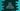# Dart break statement explanation with example# Dart break statement :

break statement is used to stop the current execution. break is used with a switch block or with loops. With switch, we need to use one break statement at the end of each case block. Once a case block is executed, it goes out of that switch block.

Similarly, we can use break with any loops. It stops the execution once it reaches one break statement.

In this tutorial, I will show you how break works with different examples.

## Break with while loop :

``````main() {
var i = 0;

while (i++ < 10) {
if (i == 6) {
break;
}
print("i = \$i");
}
}``````

This will print the below output :

``````i = 1
i = 2
i = 3
i = 4
i = 5``````

Here, we are printing the value of i inside the while loop. On each step, we are incrementing the value of i by 1. But if the value of i is equal to 6, it executes the break statement, i.e. exits from the loop.

The condition is to run the while loop until i is less than 10. But, since we are exiting the loop on i == 6, it runs only from i = 1 to i = 5.

## Break with for loop :

Similar to while, we can also use break with a for loop :

``````main() {
for (var i = 1; i < 10; i++) {
if (i == 6) {
break;
}
print("i = \$i");
}
}``````

Output :

``````i = 1
i = 2
i = 3
i = 4
i = 5``````

Same as the above example, it exits from the loop.

## Break with nested for loop :

``````main() {
for (var i = 1; i < 3; i++) {
for (var j = 1; j < 10; j++) {
if (j == 2) {
break;
}
print("i = \$i, j = \$j");
}
}
}``````

If you execute break inside an inner loop, it exits from that loop.

In the above example, the outer loop runs from i = 1 to i = 2 and the inner loop runs from j = 1 to j = 9. It will print the below output :

``````i = 1, j = 1
i = 2, j = 1``````

The outer loop runs for i = 1 and i = 2. The inner loop is defined to run from j = 1 to j = 9, but we have one break statement for j = 2. So, it will run only for j = 1 and j = 2, but it will break for j = 2 before executing the print statement. So, the output is only for j = 1.